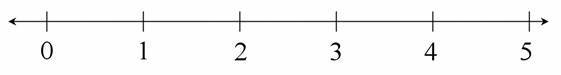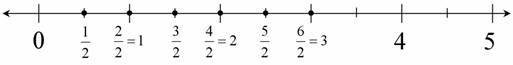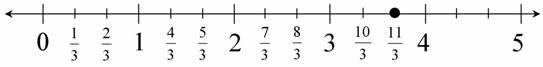Locating Fractions Greater than One on the Number Line

Alignments to Content Standards: 3.NF.A.2

1. Mark and label points on the number line for $\frac12$, $\frac22$, $\frac32$, $\frac42$, $\frac52$, and $\frac62$.2. Mark and label a point on the number line for $\frac{11}{3}$. Be as exact as possible.IM Commentary

This first part of this task requires students to represent a fraction $\frac{a}{b}$ on a number line diagram by marking off $a$ intervals of length $\frac1b$ (in this case $\frac12$) starting at 0.

Students may solve the second part of the task either by marking of 11 intervals of length $\frac13$ or by knowing that $\frac{11}{3}$ is $\frac23$ of the way between 3 and 4. Although a few students may be able to do it using the later of these approaches, most students will identify all of the points related to $\frac13$, $\frac23$, $\frac33$, etc., even if they do not label those points.

The following lists related tasks in order of sophistication:

• Locating Fractions Less than One on the Number Line
• Locating Fractions Greater than One on the Number Line
• Closest to $\frac12$
• Find 1
• Find $\frac23$
• Which is Closer to 1?

Solution

1. Here is one way to draw and label the number line for this task:2. Here is one way to draw and label the number line for this task: